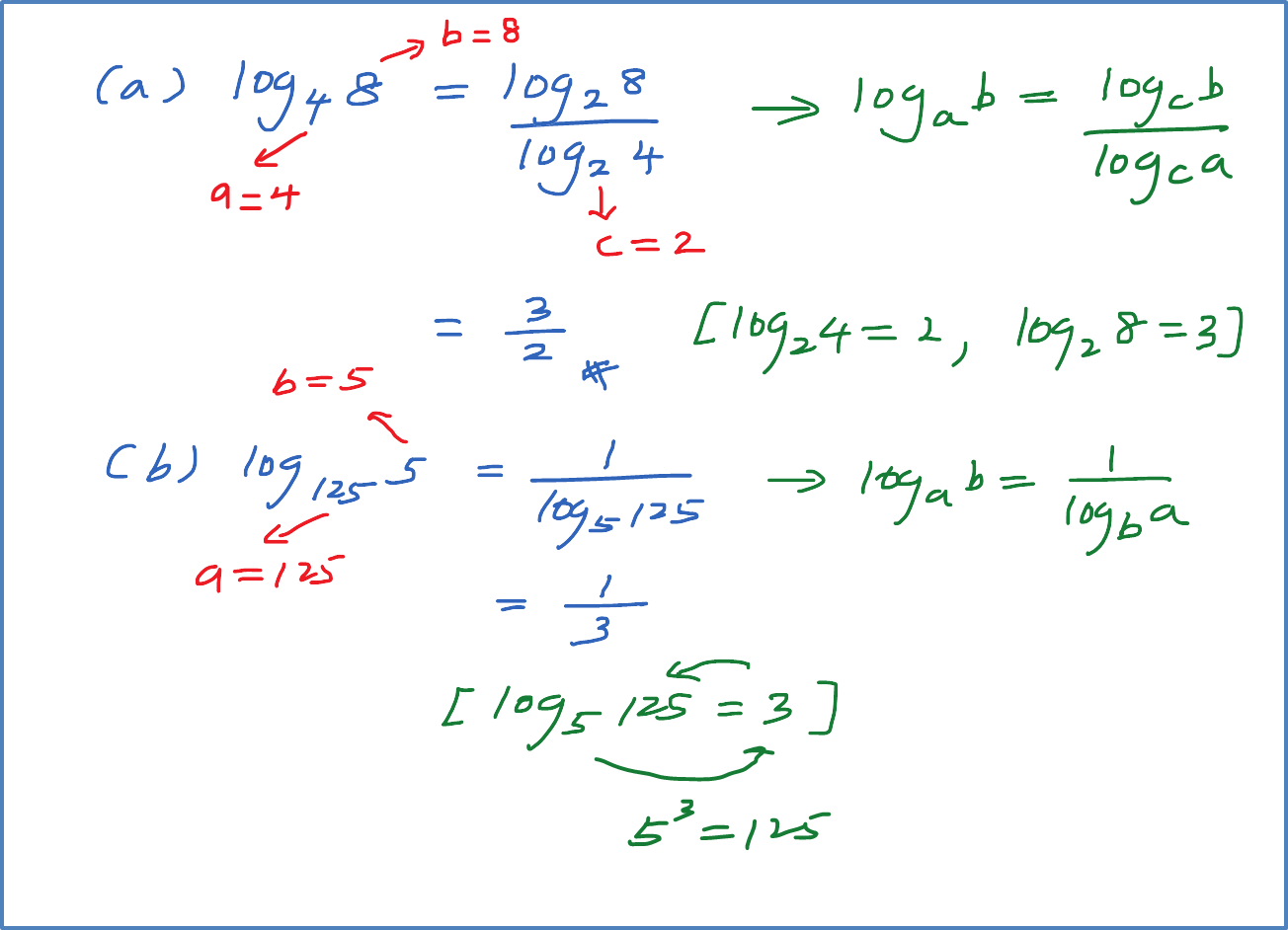# 4.2.2(a) Example 1 (Change of Base of Logarithms)

Example 1
Find the value of the following.
(a) ${\mathrm{log}}_{4}8$
(b) ${\mathrm{log}}_{125}5$
(c) ${\mathrm{log}}_{81}27$
(d) ${\mathrm{log}}_{16}64$